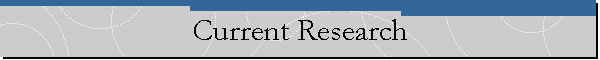In my study of a special case of rings of the form A+XB[X] where B is a quotient ring of A, I have recently shown that if A is a Prufer v-multiplication domain A+XA[1/S][X] is a Prufer v-multiplication domain (PVMD) if and only if for every nonzero d in A, the ideal (d, X) is t-invertible. Here, A is a PVMD if every finitely generated nonzero ideal I of A is t-invertible, i.e. there is a finitely generated ideal J such that A: (IJ)=A. The fall out from this study is an interesting set of results. I am writing two papers on this with Dan Anderson. The titles of these two papers are:

1. Splitting multiplicative sets . (This paper has been accepted for publication in Proc. Amer. Math. Soc.)
2. The ring R+XR[1/S][X] and t-splitting sets. This paper is still under construction.

back